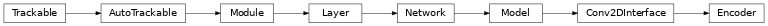# Encoder¶

Inheritance Diagramclass ashpy.models.convolutional.encoders.Encoder(layer_spec_input_res, layer_spec_target_res, kernel_size, initial_filters, filters_cap, output_shape, use_dropout=True, dropout_prob=0.3, non_linearity=<class 'tensorflow.python.keras.layers.advanced_activations.LeakyReLU'>)[source]

Primitive Model for all encoder (i.e., convolution) based architecture.

Notes

Default to DCGAN Discriminator architecture.

Examples

• Direct Usage:

dummy_generator = Encoder(
layer_spec_input_res=(64, 64),
layer_spec_target_res=(8, 8),
kernel_size=5,
initial_filters=4,
filters_cap=128,
output_shape=1,
)

• Subclassing

class DummyDiscriminator(Encoder):
def call(self, inputs, training=True):
print("Dummy Discriminator!")
# build the model using
# self._layers and inputs
return inputs

dummy_discriminator = DummyDiscriminator(
layer_spec_input_res=(64, 64),
layer_spec_target_res=(8, 8),
kernel_size=5,
initial_filters=16,
filters_cap=128,
output_shape=1,
)
dummy_discriminator(tf.zeros((1,28,28,3)))

Dummy Discriminator!


Methods

 __init__(layer_spec_input_res, …[, …]) Instantiate the Decoder.

Attributes

 activity_regularizer Optional regularizer function for the output of this layer. dtype dynamic inbound_nodes Deprecated, do NOT use! Only for compatibility with external Keras. input Retrieves the input tensor(s) of a layer. input_mask Retrieves the input mask tensor(s) of a layer. input_shape Retrieves the input shape(s) of a layer. input_spec Gets the network’s input specs. layers losses Losses which are associated with this Layer. metrics Returns the model’s metrics added using compile, add_metric APIs. metrics_names Returns the model’s display labels for all outputs. name Returns the name of this module as passed or determined in the ctor. name_scope Returns a tf.name_scope instance for this class. non_trainable_variables non_trainable_weights outbound_nodes Deprecated, do NOT use! Only for compatibility with external Keras. output Retrieves the output tensor(s) of a layer. output_mask Retrieves the output mask tensor(s) of a layer. output_shape Retrieves the output shape(s) of a layer. run_eagerly Settable attribute indicating whether the model should run eagerly. sample_weights state_updates Returns the updates from all layers that are stateful. stateful submodules Sequence of all sub-modules. trainable trainable_variables Sequence of variables owned by this module and it’s submodules. trainable_weights updates variables Returns the list of all layer variables/weights. weights Returns the list of all layer variables/weights.
__init__(layer_spec_input_res, layer_spec_target_res, kernel_size, initial_filters, filters_cap, output_shape, use_dropout=True, dropout_prob=0.3, non_linearity=<class 'tensorflow.python.keras.layers.advanced_activations.LeakyReLU'>)[source]

Instantiate the Decoder.

Parameters: layer_spec_input_res (tuple of (int, int)) – Shape of the input tensors. layer_spec_target_res (Union[int, Tuple[int, int]]) – (tuple of (int, int)): Shape of tensor desired as output of _get_layer_spec(). kernel_size (int) – Kernel used by the convolution layers. initial_filters (int) – Numbers of filters to used as a base value. filters_cap (int) – Cap filters to a set amount, in the case of an Encoder is a ceil value AKA the max amount of filters. output_shape (int) – Amount of units of the last tf.keras.layers.Dense. None ValueError – If filters_cap < initial_filters
_add_building_block(filters)[source]

Construct the core of the tf.keras.Model.

The layers specified here get added to the tf.keras.Model multiple times consuming the hyper-parameters generated in the _get_layer_spec().

Parameters: filters (int) – Number of filters to use for this iteration of the Building Block.
_add_final_block(output_shape)[source]

Prepare the results of _add_building_block() for the final output.

Parameters: output_shape (int) – Amount of units of the last tf.keras.layers.Dense